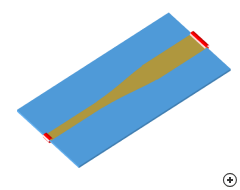Continuously tapered microstrip transitionImage of the Continuously tapered microstrip transition.

Two arbitrary real impedances may be matched over a desired bandwidth by using a multi-section matching transformer. As the number of discrete sections increases, the step change in characteristic impedance between adjacent sections becomes smaller; this, in the limit of an infinite number of sections approaches a continuous taper. The type of continuous taper determines the characteristics of the passband.

The types of tapers implemented here are Binomial and Chebyshev (multi-section quarter-wave), and Klopfenstein (sampled continuous).

The types of tapers implemented here are Klopfenstein, Hecken, Triangular, Exponential and Linear. For a given taper length, the Klopfenstein taper has been shown to achieve the lowest reflection coefficient; while for a maximum reflection coefficient specification, it has the shortest length.